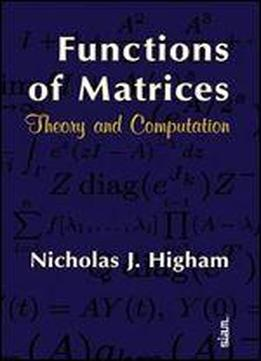# Functions Of Matrices: Theory And Computation by Nicholas J. Higham / 2008 / English / PDF

This superb book is timely and is written with great attention paid to detail, particularly in its referencing of the literature. The book has a wonderful blend of theory and code (MATLAB) so will be useful both to nonexperts and to experts in the field. Alan Laub, Professor, University of California, Los Angeles The only book devoted exclusively to matrix functions, this research monograph gives a thorough treatment of the theory of matrix functions and numerical methods for computing them. The author's elegant presentation focuses on the equivalent definitions of f(A) via the Jordan canonical form, polynomial interpolation, and the Cauchy integral formula, and features an emphasis on results of practical interest and an extensive collection of problems and solutions. Functions of Matrices: Theory and Computation is more than just a monograph on matrix functions its wide-ranging contentincluding an overview of applications, historical references, and miscellaneous results, tricks, and techniques with an f(A) connectionmakes it useful as a general reference in numerical linear algebra.Other key features of the book include development of the theory of conditioning and properties of the Frchet derivative an emphasis on the Schur decomposition, the block Parlett recurrence, and judicious use of Pad approximants the inclusion of new, unpublished research results and improved algorithms a chapter devoted to the f(A)b problem and a MATLAB toolbox providing implementations of the key algorithms.Audience: This book is for specialists in numerical analysis and applied linear algebra as well as anyone wishing to learn about the theory of matrix functions and state of the art methods for computing them. It can be used for a graduate-level course on functions of matrices and is a suitable reference for an advanced course on applied or numerical linear algebra. It is also particularly well suited for self-study. Contents: List of Figures List of Tables Preface Chapter 1: Theory of Matrix Functions Chapter 2: Applications Chapter 3: Conditioning Chapter 4: Techniques for General Functions Chapter 5: Matrix Sign Function Chapter 6: Matrix Square Root Chapter 7: Matrix pth Root Chapter 8: The Polar Decomposition Chapter 9: Schur-Parlett Algorithm Chapter 10: Matrix Exponential Chapter 11: Matrix Logarithm Chapter 12: Matrix Cosine and Sine Chapter 13: Function of Matrix Times Vector: f(A)b Chapter 14: Miscellany Appendix A: Notation Appendix B: Background: Definitions and Useful Facts Appendix C: Operation Counts Appendix D: Matrix Function Toolbox Appendix E: Solutions to Problems Bibliography Index.

views: 353